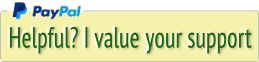## Software development, photography, jokes, ....## Sites by me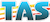Transportation Administration System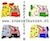Snoezelen Pillows for Dementia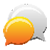Begeleiders voor gehandicapten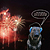Laat uw hond het jaarlijkse vuurwerk overwinnen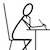Betuweroute en Kunst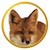Hey Vos! Je eigen naam@vos.net emailadres?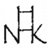Kunst in huis? Nicole Karrèr maakt echt bijzonder mooie dingen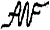Kunst in huis? Netty Franssen maakt ook bijzonder mooie dingen

## Hosting Favorites

ANU Internet Services
Netim
XEL Media

## Blogroll

Bomenstichting
PHP
MySQL
jQuery
jQuery UI
Favicon Generator.
Check HTTPS problems

`google_ad_client = "ca-pub-7184854235163319";/* marcvosnet2 */google_ad_slot = "2269232649";google_ad_width = 200;google_ad_height = 600;`

# Marc's Place

`google_ad_client = "ca-pub-7184854235163319";/* marcvosnet */google_ad_slot = "4057935566";google_ad_width = 728;google_ad_height = 90; `

# A better PHP eval()## Why not use PHP eval() directly?

`<?php/*Author : M.H.E. VosCopyright : (c) 2021, SDS82Creation date : 2021-06-16*/function mv_eval(\$p1, \$p2, \$p3) {    /*        Use this function instead of eval() directly, to prevent PHP and SQL injection.        p1 = formula for eval(). Must be in ().        p2 = array() with texts and values to look for and replace in the formula            array('myvar' => \$myvar[, ...])            or            array('\$myvar' => \$myvar[, ...])            Do not put the keys of the array between double quotes.        p3 = default value to return in case of errors. Can also be 'false'        Examples:            \$zzVAR1 = mv_eval(\$varwithformula, array('myvar' => \$myvar), false);            if(\$zzVAR1 === false) { print('Error in formula'); }            \$zzVAR1 = mv_eval(\$varwithformula, array('myvar' => \$myvar), 123);    */    if(strlen(\$p1) < 3) {        return(false);    }    if(!is_array(\$p2)) {        return(false);    }    // Look for variables in formula    \$f = \$p1;    foreach(\$p2 as \$key => \$value) {        \$f = str_replace((\$key != '\$' ? '\$' : '') . \$key, \$value, \$f);    }    // After all variables have been replaced with their real vaules, remove remaining characters, single and double quotes and semicolons.    \$f = preg_replace("/[a-zA-Z'\";]/", "", \$f);    // Remove possible leftovers: spaces and empty round brackets, for example () or ( ()).    // A PHP formula does not need spaces.    \$x = \$f;    \$f = str_replace('()', '', str_replace(' ', '', \$f));    while(\$x != \$f) {        \$x = \$f;        \$f = str_replace('()', '', str_replace(' ', '', \$f));    }    // Execute eval(). If it fails, return the default value.    try {        \$x = @eval('return(' . \$f . ');');    } catch (ParseError \$e) {        \$x = \$p3;    }    return(\$x);}?>`